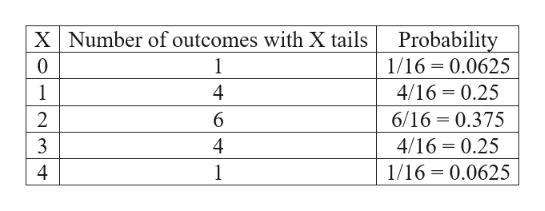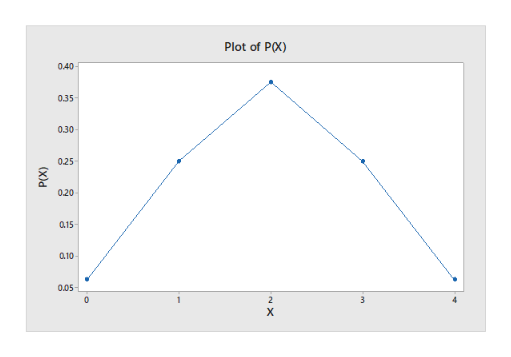# 1. A random experiment consists of throwing 4 equal coins, if X is the number of tails:a. Find the probability distribution of X and plot your graphb. What is the most likely tail number? Which one is the least likely?c. What is the average number of tails that come out, when throwing the four coins? d. What is the dispersion of X?

Question

1. A random experiment consists of throwing 4 equal coins, if X is the number of tails:

a. Find the probability distribution of X and plot your graph

b. What is the most likely tail number? Which one is the least likely?

c. What is the average number of tails that come out, when throwing the four coins? d. What is the dispersion of X?

check_circleExpert Solution
Step 1

Hey, since there are multiple subparts posted, we will answer first three subparts. If you want any specific question to be answered then please submit that question only or specify the question number in your message.”

(a)

The outcome for throwing 4 equal coins is,

HHHH, HHHT, HHTH, HHTT, HTHH, HTHT, HTTH, HTTT, THHH, THHT, THTH, THTT, TTHH, TTHT, TTTH, TTTT.

Thus, the total number of outcomes is 16.

The probability distribution of X is,help_outlineImage TranscriptioncloseXNumber of outcomes with X tails Probability 1/16 0.0625 0 4 4/16 0.25 1 6/16 0.375 2 6 4/16 0.25 4 1/16 0.0625 4 1 fullscreen
Step 2

Step by step procedure to plot the graph using MINITAB software is given below:

• Choose Graph > Time Series Plot or Stat > Time Series > Time Series Plot.
• Choose Simple, and then click OK.
• In Series, enter P(X).
• In Time and Scale, enter X under Stamp.
• Click OK.

Output using the MINITAB software is given below:help_outlineImage TranscriptionclosePlot of PX) 0.40 0.35 0.30 025 020 a15 0.10 0,05 0 2 3 х (x)d fullscreen
Step 3

(b)

From the plot, it is observed that the most likely tail number is 2, because the probability value is greater for X=2 and the least likely is 0 and 4 because the probability value is lesser when compare...

### Want to see the full answer?

See Solution

#### Want to see this answer and more?

Solutions are written by subject experts who are available 24/7. Questions are typically answered within 1 hour*

See Solution
*Response times may vary by subject and question
Tagged in

### Other